# pylops.Laplacian#

class pylops.Laplacian(dims, axes=(-2, -1), weights=(1, 1), sampling=(1, 1), edge=False, kind='centered', dtype='float64', name='L')[source]#

Laplacian.

Apply second-order centered Laplacian operator to a multi-dimensional array.

Note

At least 2 dimensions are required, use pylops.SecondDerivative for 1d arrays.

Parameters
dimstuple

Number of samples for each dimension.

axesint, optional

New in version 2.0.0.

Axes along which the Laplacian is applied.

weightstuple, optional

Weight to apply to each direction (real laplacian operator if weights=(1, 1))

samplingtuple, optional

Sampling steps for each direction

edgebool, optional

Use reduced order derivative at edges (True) or ignore them (False) for centered derivative

kindstr, optional

Derivative kind (forward, centered, or backward)

dtypestr, optional

Type of elements in input array.

Raises
ValueError

If axes. weights, and sampling do not have the same size

Notes

The Laplacian operator applies a second derivative along two directions of a multi-dimensional array.

For simplicity, given a two dimensional array, the Laplacian is:

$y[i, j] = (x[i+1, j] + x[i-1, j] + x[i, j-1] +x[i, j+1] - 4x[i, j]) / (\Delta x \Delta y)$

Methods

 __init__(dims[, axes, weights, sampling, ...]) adjoint() apply_columns(cols) Apply subset of columns of operator cond([uselobpcg]) Condition number of linear operator. conj() Complex conjugate operator div(y[, niter, densesolver]) Solve the linear problem $$\mathbf{y}=\mathbf{A}\mathbf{x}$$. dot(x) Matrix-matrix or matrix-vector multiplication. eigs([neigs, symmetric, niter, uselobpcg]) Most significant eigenvalues of linear operator. matmat(X) Matrix-matrix multiplication. matvec(x) Matrix-vector multiplication. reset_count() Reset counters rmatmat(X) Matrix-matrix multiplication. rmatvec(x) Adjoint matrix-vector multiplication. todense([backend]) Return dense matrix. toimag([forw, adj]) Imag operator toreal([forw, adj]) Real operator tosparse() Return sparse matrix. trace([neval, method, backend]) Trace of linear operator. transpose()

## Examples using pylops.Laplacian#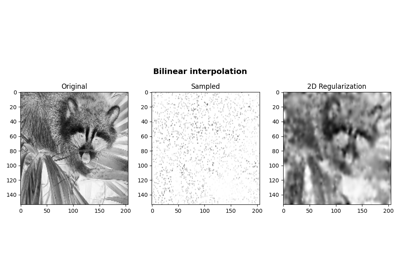Bilinear Interpolation

Bilinear Interpolation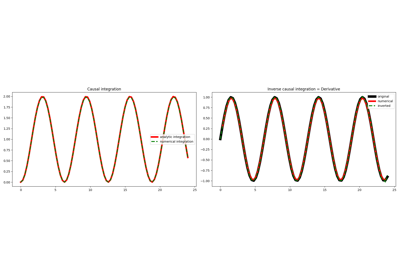Causal Integration

Causal Integration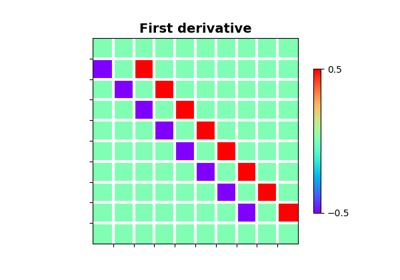Derivatives

Derivatives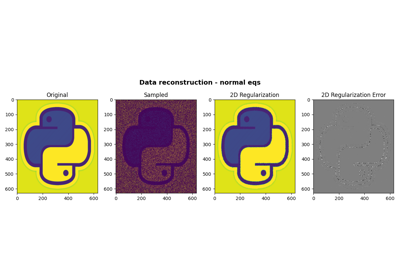06. 2D Interpolation

06. 2D Interpolation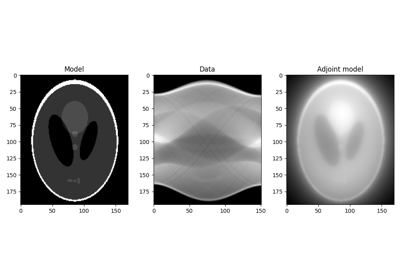16. CT Scan Imaging

16. CT Scan Imaging